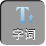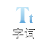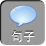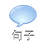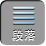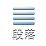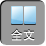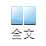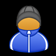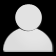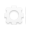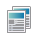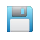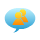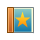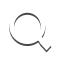-AA+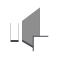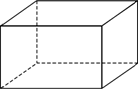1、下列方程中，是一元一次方程的是（）

A. 2 x3 y5

B. x 2x20

C. 3 x54 x1

D.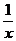x1

2、方程（ m1x| m|10是关于 x的一元一次方程，则（）

A. m＝± 1

B. m1

C. m＝－ 1

D. m≠－ 1

3、检验下列各数是不是方程 5 x272 x的解，并写出检验过程.

1x2；（ 2x3.

2）将 x3代入方程，左边＝ 13，右边＝ 13，左边＝右边，故 x3是方程 5 x272 x的解.

4、某文具店一支铅笔的售价为 1.2元，一支圆珠笔的售价为 2元.该店在“ 6· 1儿童节”举行文具优惠售卖活动，铅笔按原价打 8折出售，圆珠笔按原价打 9折出售，结果两种笔共卖出 60支，卖得金额 87元.若设铅笔卖出 x支，则依题意可列得的一元一次方程为（）

A. 1.2× 0.8 x2× 0.960x）＝ 87

B. 1.2× 0.8 x2× 0.960x）＝ 87

C. 2× 0.9 x1.2× 0.860x）＝ 87

D. 2× 0.9 x1.2× 0.860x）＝ 87

5、已知 mn，则下列等式不成立的是（）

A. m1n1

B.2 m1＝－ 12 n

C.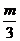1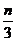1

D. 23 m3 n2

6、用等式的性质解下列方程：

14 x73；（ 2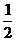x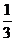x4.

2）方程两边都乘以 6，得 3 x2 x24x24.

1.下列各式，是一元一次方程的有 （ ）

x= 3；② x+ y= 6；③ x 2+ 3 x= 1；④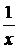=－ 2；⑤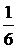x=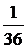；⑥ 2 x3

x7= 10.

A. 3

B. 4

C. 5

D. 6

2.x= 1是方程 2 xa= 0的解，则 2 a=（）

A. 1

B.1

C. 4

D.4

3.吴悦买书需用 48元钱，付款时恰好用了 1元和 5元的纸币共 12张.设所用的 1元纸币为 x张，根据题意，下面所列方程正确的是（）

A. x+ 512x）= 48

B. x+ 5x12）= 48

C. x+ 12x5）= 48

D. 5 x+（ 12x）= 48

4.如图，如果天平右盘里放了一块砖，左盘里放了半块砖和 2 kg的砝码，天平两端正好平衡，那么一块砖的质量是（）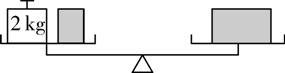A. 1 kg

B. 2 kg

C. 3 kg

D. 4 kg

5.下列说法正确的有（ ）

①若 3 x+ 2= 7，则 3 x= 72；②若 3 ax= 3 ay，则 x= y；③若 x+ 3 y= 3 y+ ANOAHDIGITAL 10，则 x= ANOAHDIGITAL 11；④若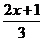=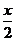，则 22 x+ 1）= 3 x；⑤等式两边同时除以同一个数，所得结果仍是等式. （ ）

A. 1

B. 2

C. 3

D. 4

6.已知等式 3 a= 2 b+ 5，则下列等式不一定成立的是（）

A. 3 a5= 2 b

B. 3 a+ 1= 2 b+ 6

C. 3 ac= 2 bc+ 5

D. a=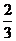b+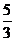7.张明的妈妈今年 44岁，她的年龄比张明年龄的 3倍还大 2岁，设张明今年 x岁，则可列方程为______.

8.已知 x= 2是方程 3 x1= 2 x+ m的解，求 m的值.

9.根据题意设未知数并列出方程（不必求解）

1）已知长方形的周长是 16 cm，长比宽多 2 cm，则这个长方形的长是多少？

2）把若干本书发给学生，如果每人发 4本，还剩下 2本；如果每人发 5本，还差 5本，那么共有多少名学生？

1. A解析：判断一个方程是不是一元一次方程必须满足：①是整式方程，②只含有一个未知数，③未知数的指数为 1，④未知数的系数不等于 0，四者缺一不可.所以①⑤⑦是一元一次方程故选 A.

2. C解析：将 x= 1代入 2 xa= 0，得 2a= 0，解得 a= 2，则 2 a= 2× 2= ANOAHDIGITAL 10.故选 C.

3. A

4. D解析：设一块砖的质量是 x kg，则根据题意，得x+ 2= x.利用等式的基本性质解得 x= 4.故一块砖的质量是 4 kg.故选 D.

5. C解析：利用等式的基本性质对方程进行恒等变形.说法正确的是①③④，共 3个.故选 C.

6. C解析：利用等式的基本性质对方程进行恒等变形.选项 C，等式右边的 5没有乘 c，所以不成立.故选 C.

7. 3 x+ 2= 44解析：先找出等量关系“今年张明的妈妈的年龄比张明年龄的 3倍还大 2岁”，再列方程.

8.解：因为 x= 2是方程 3 x1= 2 x+ m的解，所以将 x= 2代入方程，得

3× 212× 2+ m

9.解：（ 1）设这个长方形的长是 x cm，则宽是（ x2cm.由题意，得 2 x+ 2x2）= 16.

2）设共有 x名学生.